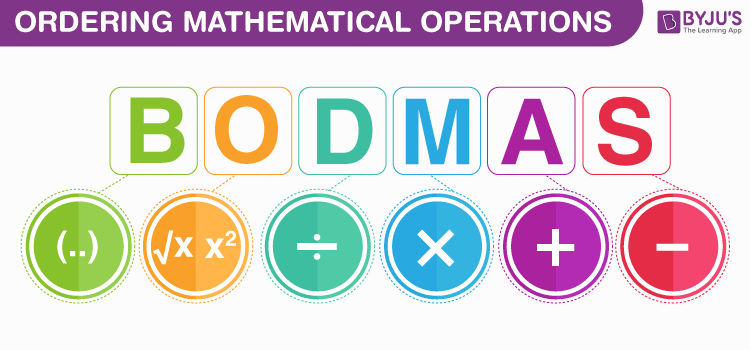# What is BODMAS ?

The evaluation of an expression involving numbers is one of the basic tasks in arithmetic. If an expression contains addition (+), subtraction (-), multiplication (x), division (÷), bracket (), and Of (of), then the precedence of simplification of the operation is given by the “BODMAS rule”. First, we have to know what is BODMAS rule? Or What is the BODMAS formula?

BODMAS rule is a rule or order that is used to simplify the arithmetic expression involving more operators in mathematics. When an equation involves more operators, first, we have to know in which order we have to solve the expression. This problem is solved with the help of the BODMAS rule.The full form of BODMAS is:

• Brackets (Parts of a calculation inside the bracket always come first).
Precedence of brackets are : [{(bar)}]
• Orders (powers and square roots)
• Division
• Multiplication
• Subtraction

Each letter of the word stands for the first letter of an operator. If an expression involves two or more similar operators that appear in succession, then the precedence is from left to right. By using BODMAS, we can evaluate an expression in the correct order of precedence.

Anything inside the bracket is done first. Then evaluate any powers or roots. Next, do any division or multiplication in an expression working from left to right and then followed by addition and subtraction again from left to right. Here, division and multiplication have the same priority and addition and subtraction have the same priority.

## What is BODMAS Rule in Maths?

Mathematics is a logic-based discipline. In arithmetic, an expression or an equation involves two components, namely,

• Numbers – A number is a mathematical value which is used for representing a particular quantity and it is used in counting and making calculations. The symbol that represents a number is called a numeral. According to the property, numbers can be classified as
1. Natural Numbers
2. Integers
3. Rational Numbers
4. Irrational Numbers
5. Real Numbers
6. Complex Numbers
7. Imaginary Numbers
• Operators or operations: An operator is a character which combines two numbers and produces an expression or equation. The most common operators used in mathematics are :
2. Subtraction (-)
3. Multiplication (x)
4. Division (÷)

In mathematics, the order of operations or the operator precedence is given by the BODMAS rule. The symbol used for grouping the value is a bar. Grouped symbols are treated as a single expression.

## Solved Problems

Problems based on the BODMAS rule involving integers and decimals values are given below

Question 1 :

Find the value of 2[2+2{39-2(17+2)}]

Solution :

Given, 2[2+2{39-2(17+2)}]

By using BODMAS Rule,

First, simplify the value inside the brackets ()

= 2[2+2{39-2(19)}]

= 2[2+2{39-38}]

subtract the value inside the brackets {}

= 2[2+2{1}]

= 2[2+2]

Add the value inside the brackets [ ]

=2

Finally, multiply both values, which gives 8.

Therefore, the value of 2[2+2{39-2(17+2)}] is 8

Question 2 :

Find the value of 4.5 – [2 + 0.5 of (2.1 – 1.3 x 1.01)]

Solution:

Given, 4.5 – [2 + 0.5 of (2.1 – 1.3 x 1.01)]

By using BODMAS Rule,

First simplify the value inside the brackets ()

= 4.5 – [2 + 0.5 of (2.1 – 1.3 x 1.01)]             “Multiply 1.3 x 1.01”

= 4.5 – [2 + 0.5 of 0.787]                               “Subtract 1.313 from 2.1”

Simplify the value inside the brackets [ ]

= 4.5 – [2 + 0.3935]                                      “Multiply 0.5 x 0.787”

= 4.5 – 2.3935                                                 “Add two values inside the brackets [ ]”

Finally, Subtract the values

= 2.1065

Therefore, the value of 4.5 – [2 + 0.5 of (2.1 – 1.3 x 1.01)] is 2.1065.

Test your Knowledge on What is BODMAS